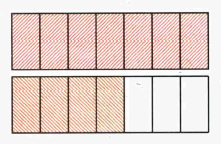# Mixed Numbers/Fractions

Mixed number (or mixed fraction) is a number that consists of integer and proper fraction.

In other words mixed numbers are "mix" of integer and proper fraction.

We write it in the following way: ${4}\frac{{1}}{{5}}$. Here, 4 is integer and $\frac{{1}}{{5}}$ is proper fraction.

Other examples of mixed numbers are ${15}\frac{{7}}{{9}}$, ${45}\frac{{15}}{{16}}$ etc.

Note, that, for example, ${14}\frac{{8}}{{7}}$ is not correct mixed number, because $\frac{{8}}{{7}}$ is not proper fraction.Another example of mixed numbers is following.

Suppose you have two rectangles. You divide each of them into 7 parts. You paint all 7 parts of first rectangle, i.e. you paint all rectangle and 4 parts of second rectangle.

In this case you paint ${1}\frac{{4}}{{7}}$.

In fact, mixed number is sum of integer and proper fraction: ${1}+\frac{{4}}{{7}}$. We will see later how to perform such addition, but for now remember that ${1}\frac{{4}}{{7}}$ is equivalent to ${1}+\frac{{4}}{{7}}$.